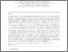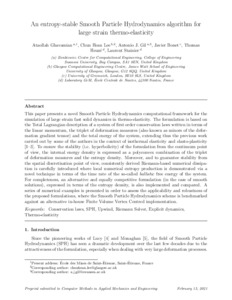# An entropy-stable Smooth Particle Hydrodynamics algorithm for large strain thermo-elasticity

Ghavamian, Ataollah, Lee, Chun Hean, Gil, Antonio J., , Heuzé, Thomas and (2021) An entropy-stable Smooth Particle Hydrodynamics algorithm for large strain thermo-elasticity. Computer Methods in Applied Mechanics and Engineering, 379:113736. ISSN 0045-7825 (doi:https://doi.org/10.1016/j.cma.2021.113736)Preview
PDF (Author Accepted Manuscript)
31838 BONET_An_Entropy-Stable_Smooth_Particle_(AAM)_2021.pdf - Accepted Version

## Abstract

This paper presents a novel Smooth Particle Hydrodynamics computational framework for the simulation of large strain fast solid dynamics in thermo-elasticity. The formulation is based on the Total Lagrangian description of a system of first order conservation laws written in terms of the linear momentum, the triplet of deformation measures (also known as minors of the deformation gradient tensor) and the total energy of the system, extending thus the previous work carried out by some of the authors in the context of isothermal elasticity and elasto-plasticity [1-3]. To ensure the stability (i.e. hyperbolicity) of the formulation from the continuum point of view, the internal energy density is expressed as a polyconvex combination of the triplet of deformation measures and the entropy density. Moreover, and to guarantee stability from the spatial discretisation point of view, consistently derived Riemann-based numerical dissipation is carefully introduced where local numerical entropy production is demonstrated via a novel technique in terms of the time rate of the so-called ballistic free energy of the system. For completeness, an alternative and equally competitive formulation (in the case of smooth solutions), expressed in terms of the entropy density, is also implemented and compared. A series of numerical examples is presented in order to assess the applicability and robustness of the proposed formulations, where the Smooth Particle Hydrodynamics scheme is benchmarked against an alternative in-house Finite Volume Vertex Centred implementation.

Item Type: Article Conservation laws, SPH, Upwind, Riemann Solver, Explicit dynamics, Thermo-elasticity T Technology > TA Engineering (General). Civil engineering (General) Vice-Chancellor's Group 10 Mar 2022 01:38 http://gala.gre.ac.uk/id/eprint/31838View Item